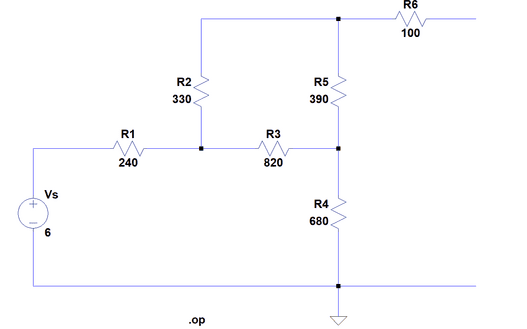# I need help finding the Thevenin equivalent resistance

SpiralStars

## Homework Statement

https://imgur.com/a/XD3Km4tI'm trying to find the thevenin equivalent resistance across the opened terminals.

## Homework Equations

I know you short circuit the voltage source and do simple circuit analysis, however i'm getting confused in determining what resistors are parallel to each other and how to redraw the diagram once I simplified. How do I do this ?

## The Attempt at a Solution

My attempt got me 426.0.26 ohmz.

#### Attachments

Last edited by a moderator:

Mentor
My attempt got me 426.0.26 ohmz.

SpiralStars
https://imgur.com/a/9MyvuGK
https://imgur.com/a/AjLxpwt
this is what i did I'm not sure how to simply the circuit and redraw it.

Mentor
Hint: If there are no obvious resistances in parallel or in series that would allow you to reduce the circuit by simple methods then you should consider placing a voltage source at the "output" and solving for the current it would drive into the circuit; The problem then becomes one of general circuit analysis involving whatever methods you've learned. Once you know that current, then Ohm's law will tell you the resistance that the source sees.

•berkeman
Gold Member
The pictures are blurry but theres a approach that I always use.Lets suppose you started from one of the open terminals, lets say upper one. Also I didnt understand why you included ##100Ω## resistor.

In any case try to follow a current path in your mind. If the path divides two and you cant reach the B in 1 path then they must be parallel.

Second approach is that try to look the circuit from the open ends side but not from the normal image. I mean move the image 90 degrees right and try to look it that way. Also I think this is a homework question